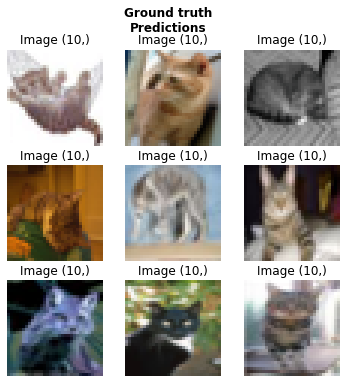hi，i have a question about inference,my dataset is define by :
data = (SegmentationItemList.from_folder(img_dir_path)
.split_by_files(val_list)
.label_from_func(get_y_fn, classes=category)
.transform(get_transforms(), tfm_y=True, size=400)
.databunch(bs=2, path=dataset_path, num_workers=6)
.normalize())
when i inference, i got the result size 400*400,that is not strange by what your explanation,(For inference, it applies the transforms you had set on the validation set),what i want to know is how to set train set transforms and validation set transforms respectively.
i seem the transforms do not apply to the validation set except the size, FCN should run with any size, i don’t want to resize src to inference then resize.what should i do to let the export model to inference picture in any size without resize to 400?
thank you.

In your call to `transform`, you pass a tuple of list of transforms (the return from `get_transforms()`). First list is for the training set, second list for the validation/test set.

Thank you very much.
The parameter of size seems not in the tfms list .Could it work for any size of input picture without resize?
When train the model I want to resize the input,but when i test and verify I want it could keep the input size.What can i do?

You can’t batch images if they are not of the same size.

I do test and val without batch. Just one by one. Or you can think that there is not validation set in my model. I just train and then predict(I will computer the metrics by myself). What can i do to set the dataset?

Just create a different databunch for your test data then. There is no point having it in the same databunch as the training set since you don’t want to validate on it.

I get it.Thank you very much!

You can pass `tfm_y=False` when adding your test data to avoid having those transforms (like resize) applied there but your predictions won’t match your original images (if you resize for instance, they will have the resized size).

Just to clarify, I don’t think this approach works with `load_learner()`.

I have tried the following:

``````test_src = SegmentationItemList.from_df(test, "myPath/test", cols='im_id')
``````

FastAI still tries to apply transforms to `EmptyLabelList`.

What’s happening is:

1. I call `load_learner()`
2. `load_learner()` creates a `LabelLists` called `src` with train/valid settings inherited from training
3. `load_learner()` calls `add_test()` (Notably we cannot pass in `tfm_y` here)
4. `add_test()` creates a test set from our valid set
5. When creating the test, `tfm_y` is None, so we default to the `valid` `tfm_y` which is True

The end result is that we end up trying to apply transforms to the test set.

If I copy and paste `load_learner` into my own project and modify the call to `add_test()` to include `tfm_y=False` it seems to work as I would expect.

1 Like

Yes, that should be an argument in the signature of `load_learner`. You should make a PR with that change.

Sounds good, I’ll make it later today.

A related question: Why after using load_learner to load an exported trained model, GPU memory is consumed (I am doing inference on my GPU server for now for my experiments)? Is there a way to impose CPU utilization when calling load_learner?
Thank you

There is a flag to force-load your model on the CPU, by default it loads it on the same kind of device as it was saved,

1 Like

If I may also ask an additional question: according to this post: Load_learner on CPU throws "RuntimeError('Attempting to deserialize object on a CUDA)" there is no more a cpu argument (like the one described in the docs: https://docs.fast.ai/basic_train.html#load_learner ), so what is the appropriate way to set this flag?

Oh indeed, you have to change `defaults.device` to `torch.device('cpu')`.

1 Like

fastai version: 1.0.60.dev0

I try to display test set images with proper predicted labels:

``````learn = load_learner(modelpath, file='resnet34-acc.82775',
test=ImageList.from_folder(path/'test'))
preds = learn.TTA(ds_type=DatasetType.Test)
learn.show_results(rows=3,ds_type=DatasetType.Test,figsize=(6,6))
``````

renders the following imageQuesions:

1. Image(10,) title – a bug or my fault?

2. Is there a way to show/specify predicted labels?

You can’t use show_results with a test dataset, that has no labels.

Has anyone figured out the way to load an unlabled segmentation dataset with `load_learner` without triggering the tfm_y assertion error?

I am using
`learn = load_learner(path_learner, 'export.pkl', test = SegmentationItemList.from_folder(\$TEST_DIR))`

Can I not add a test set using `load_learner`? Because I’m trying to put the model into production, and would rather not set up the whole databunch and learner if possible, since load_learner is much faster

Right now I am using

``````for i in range(curr):
img = open_image(f'{test_dir}/{i}.png')
img.resize(128)
If you check the source code of `load_learner`, it does not store the model’s name – parameter `file` in the Learner object. However, YOU can!
``````learn = load_learner(modelpath, file='resnet34-acc.82775', test=ImageList.from_folder(path/'test'))